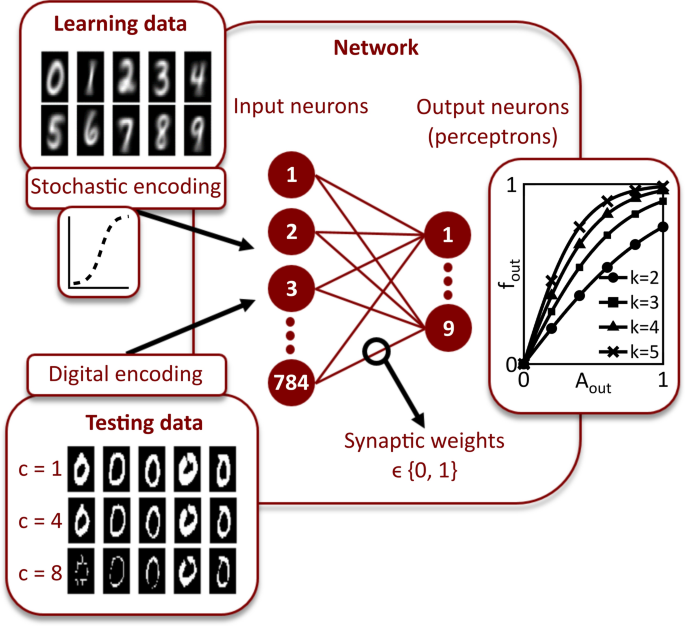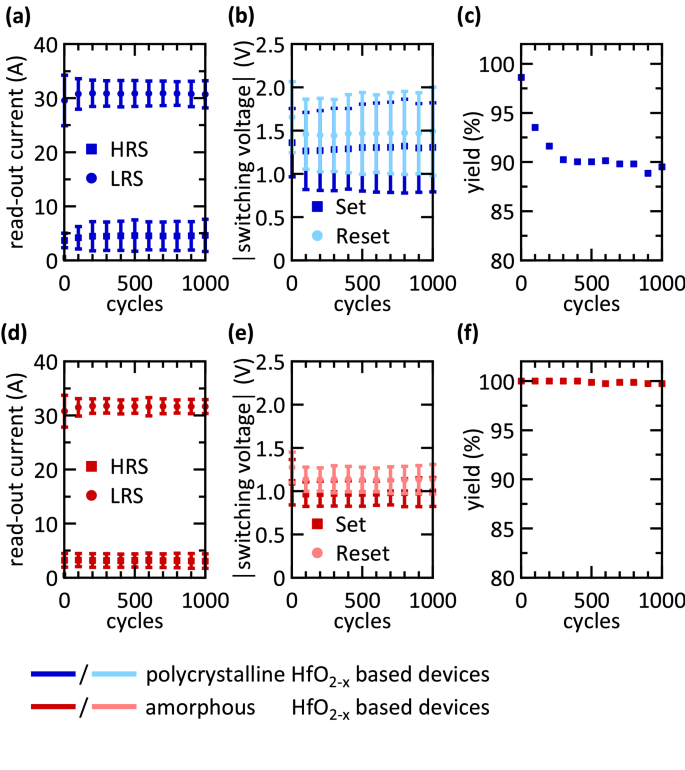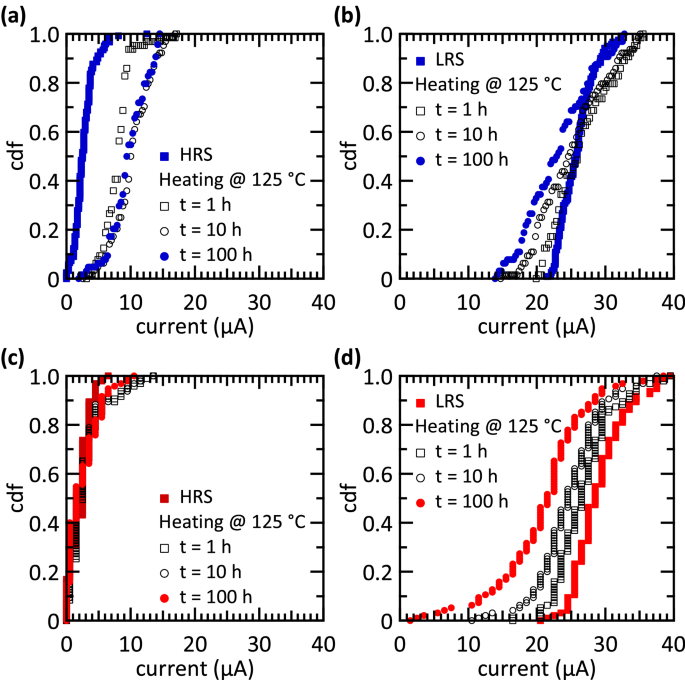# Analogue pattern recognition with stochastic switching binary CMOS-integrated memristive devices[ad_1]

### Emulation of synaptic plasticity

The inherent randomness of switching RRAM devices is employed as a plasticity model according to Ref.47. Fully CMOS-integrated 4 kbit RRAM arrays are used in a 1-transistor-1-resistor (1T-1R) configuration54,56. These cells are layered with TiN as bottom electrode, HfO2−x/TiO2−y bilayer as active layers, and TiN as top electrode. The devices possess binary resistance states, i.e. an HRS and a LRS. Before operating the devices, an effective electroforming step is required. Therefore, the incremental step pulse with verify algorithm (ISPVA)59,60 is used. Resistive switching occurs stochastically through the formation and rupturing of conductive filaments consisting of oxygen vacancies56,61. The switching to LRS, i.e. the formation of the conductive filament, is caused by the hopping of charged vacancies, which are then reduced at the filament, thereby becoming immobile61. The dissolution of the filament leads to switching to the HRS and is achieved by applying a voltage of opposite polarity. Joule heating and the electric field lead to oxidation of the vacancies and a subsequent drift of the charged vacancies. As a result, the diameter of the filament is thinned out and the filament gets ruptured61. In Ref.62 the reset process is also discussed in terms of thermo-electrochemical effects of Joule heating and ion mobility. The reset transition proceeds in gradual resistance changes, covering a limited resistance window.

According to Ref.40,47, the switching probability of applied voltage pulses can be described by a Poisson distribution, where the voltage amplitude and pulse width are taken into account. The randomness is predictable and the distribution function for a set of N voltage pulses (neural activity level) with a voltage pulse amplitude V can be written as40

$$f_{N} = frac{1}{{1 + e^{{ – dleft( {V – V_{0} } right)}} }},$$

(1)

where V0 is the threshold voltage at which the probability fN is equal to 0.5 and d is a measure of the slope of the distribution function and therefore of the switching variability. Thus, the steeper the slope, the larger the absolute value of the parameter d, and the smaller is the switching window ΔVsw in which a stochastic encoding of analogue data is possible. The switching window is defined as the voltage range in which the switching probability ({f}_{N}) is between 2 and 98%.

The device-to-device (D2D) variability of 128 1T-1R devices is evaluated by applying single voltage pulses in the set and reset regime. The switching probabilities, and thus the switching windows ΔVsw, as function of single voltage amplitudes of two different types of RRAM cells are illustrated in Fig. 1. As shown in Fig. 1(a, b) the D2D variability of the polycrystalline HfO2−x based devices is larger than the one for amorphous hafnium oxide layers (Fig. 1(c, d)). The grain boundaries of the polycrystalline HfO2−x films are causing a large device-to-device variability63. To set the devices from their inertial HRS to the LRS, a positive voltage pulse is applied to the top electrode (Fig. 1(a, c)), while a negative voltage pulse is used to reset the devices back to the HRS (Fig. 1(b, d)). The resistance states are measured at a read voltage of 0.2 V. A threshold current of 20 µA has to be exceeded for a successful set operation, while the read-out current has to be lower than 5 µA to ensure a successful reset operation. The measured data are depicted as dots in Fig. 1, while solid lines represent the distribution function according to Eq. 1, which contains the parameters d and V0. The switching windows ΔVsw are given in Fig. 1 as well. Since the switching processes are based on ion hopping and diffusion, they are stochastically by nature39,64. Thus, also a variability occurs between different cycles on one and the same device. This cycle-to-cycle (C2C) variability is shown to differ not significantly from the D2D variability in similar devices64. Furthermore, the switching voltages measured here show no correlation with the position of the devices within the 4 kbit array. Thus, using the D2D variability as a measure for stochastic switching is reasonable.Figure 1

The probability function of the set operation is equal to 0.5 at V0 = 1.04 V for polycrystalline devices, and at V0 = 0.82 V for amorphous devices. For the reset transition, this value is obtained at V0 = − 1.24 V for the polycrystalline devices, and V0 = − 1.07 V for the amorphous devices. Switching variabilities determined as d = 10.71 V−1 and d = 19.89 V−1 are obtained for the set transition for the polycrystalline and amorphous devices, respectively (Fig. 1(a, c)), while for the reset transition d = − 5.85 V−1 is measured for the polycrystalline devices, and d = − 11.41 V−1, for the amorphous ones (Fig. 1(b, d)). Thus, the variability in the reset is larger than that of the set process. Consequently, the smallest switching window is observed for the set transition of the amorphous devices (0.39 V), followed by the reset transition of the amorphous devices (0.68 V), the set transition of the polycrystalline devices (0.72 V), and the reset transition of the polycrystalline devices (1.33 V). Hence, the amorphous devices depict a lower variability than the polycrystalline devices for the same switching direction. Additionally, the absolute value of the median switching voltage V0 is lower for the amorphous devices compared to the same switching direction for the polycrystalline devices.

The higher device-to-device and cycle-to-cycle variability of the polycrystalline-HfO2 structures might be attributed to the grain boundaries conduction mechanism in polycrystalline-HfO2 structures57. The higher defect concentration leads to a higher conductivity along the grain boundaries. Furthermore, the cycle stability is affected by thermally activated diffusion of the defects from the grain boundaries. Inversely, the defect concentration in the amorphous hafnium oxide is more homogeneous distributed.

To emulate synaptic plasticity, voltage pulses with amplitudes within the switching windows are applied to the devices. Therefore, the activity A of a neuron is encoded in a voltage pulse amplitude according to

$$V = V_{1} + A cdot Delta V$$

(2)

where

$$A = frac{N}{Delta t}$$

(3)

here N is the number of action potentials arriving at a neuron in the time interval Δt, while V1 is the lower bound of the switching window. By optimising ΔV, the whole switching window can be exploited to map the activities of the neurons into voltage pulse amplitudes. This allows the mapping of analogue data to the stochastic nature of the binary memristive cells. Therefore, the influence of the switching window range on the learning performance of the network needs to be well understood, and is investigated in depth in the “Results” section of the paper.

### Network structure: stochastic artificial neural network (StochANN)

For pattern recognition a two-layer feed forward network is employed, as sketched in Fig. 2. In this configuration, every input layer neuron is connected to every output layer neuron by a memristive device to enable stochastic plasticity according to the computation scheme proposed in Ref.47. We want to emphasize, that the learning algorithm exploited in this work is emulating LTP and LTD in biology by implementing a local stochastic learning rule, which differs from conventional learning algorithms for artificial neural networks using the delta rule. For the experimental implementation a mixed-signal circuit board that couples software neurons to hardware synapses is designed. The synapses consist of RRAM devices integrated in a fully CMOS 1T-1R configuration (see “Methods”).Figure 2

Handwritten digits from the MNIST data base58 are used as input patterns. Each learning set consists of 60,000 digits from 250 different writers, and each digit is stored in a 256-level 28 × 28 pixels greyscale image. We use averaged images, which are obtained by combining 100 randomly chosen representations of each pattern and calculating the average greyscale value of the pixels. During learning, the pixel intensities pi,j of every image i are normalised within the interval [0,1] by dividing the values of every pixel j by the maximum value pi,max of the respective image

$$p_{i,j,norm} = frac{{p_{i,j} }}{{p_{i,max} }} .$$

(4)

The input images are shown in Fig. 2. These images are rearranged into a 784-row input vector so that each pixel corresponds to one input neuron, while the pixel intensities are encoded by these neurons into switching probabilities. A supervised learning algorithm is employed, where every pattern is assigned to one specific output neuron. The devices that connect the input neurons to the specific output neuron form a receptive field, while the particular resistance values are adjusted during learning. Since binary memristive devices are used, the resistance values are either in the LRS or in the HRS. To enable editing of the grey value images with these binary devices, the normalised pixel intensities pi,j,norm of the input patterns are encoded into voltage pulses. The amplitudes of these pulses represent the switching probabilities, according to Eq. 1. Either the set or the reset transitions of both technologies are used for learning. To avoid saturation effects, a low amplitude reset pulse is applied to each synaptic device if the learning is done with the set transition, while a low amplitude set pulse is employed if the learning is done with the reset transition. These voltage pulses are applied during each learning iteration.

After learning, the network performance is evaluated using the MNIST test data set containing 10,000 additional digits that differ from the ones previously used. From these data set only 50 representations of each digit are used in the experiment, while the whole data set is exploited in the simulations. Before applying these patterns to the network, their pixels are digitised to 0 or 1 obtaining binary pixel values pi,j,bin. For this purpose, a threshold Θi is determined for each test pattern according to:

$$Theta_{i} = c cdot p_{i,mean} ,$$

(5)

where pi,mean is the mean pixel value of pattern i and c is a positive constant that regulates the number of bright pixels (Fig. 2, bottom left window). Every test image is applied once to the network. As a result, the pixel intensities encoded by the input neurons are weighted through the receptive fields, which leads to a characteristic activation of the output neurons. The output neurons behaviour is reproduced with a perceptron model that exploits the activation function

$$f_{out} = frac{{1 – e^{{ – k cdot A_{out,i,m} }} }}{{1 + e^{{ – k cdot A_{out,i,m} }} }},$$

(6)

where k is a positive constant that defines the slope and Aout,i,m is the normalised activity of the input neurons for the test image i weighted by the synaptic connections wj,m to the output neuron m according to

$$A_{out,i,m} = frac{1}{784} cdot mathop sum limits_{j = 1}^{784} p_{i,j,bin} cdot w_{j,m} .$$

(7)

Therefore, the output neuron whose receptive field best corresponds to the test image shows the highest activation, and associates the test image to the pattern it learned. If several output neurons depict the same pattern, the sums of all activation functions corresponding to the same patterns are evaluated. After all test images are applied to the network, a recognition rate is determined to evaluate the accuracy of the test.

### Investigation of device variabilities

The aforementioned neural computation scheme is inherent to the stochasticity of the memristive devices. The endurance, yield, and retention of the RRAM cells can be used to assess their potential for StochANN. In this context, a closer look at the differences between the polycrystalline and amorphous memristive devices is of great relevance.

Figure 3(a, d) show the evolution of the read-out current over 1,000 switching cycles at a read-out voltage of 0.2 V. The mean values of the HRS and LRS read-out currents remain almost constant over 1,000 cycles, attesting to a high endurance. The standard deviation, illustrated by the error bars, increases during the first 200 cycles for the HRS of the polycrystalline devices. In general, the standard deviation is larger for the polycrystalline devices than for the amorphous devices. Nevertheless, the two resistance states are clearly distinguishable for both types of devices. Figure 3(b, e) present the evolution of the absolute values of the set and reset voltages for both types of devices. Again, the standard deviation of the switching voltages is larger for the polycrystalline devices than for the amorphous devices. For both sets of devices, the mean switching voltages decrease within the first 100 switching cycles and remain constant afterwards.Figure 3

The yields of the polycrystalline and amorphous devices during 1,000 cycles are shown in Fig. 3(c, f), respectively. Right after electroforming, more than 98% of the polycrystalline and 100% of the amorphous devices are able to switch. The yield of the polycrystalline devices decreases to 93.5% after 100 switching cycles, and to 89.5% after 1,000 switching cycles. In contrast, the yield of the amorphous devices only decreases to 99% after 1,000 switching cycles. For most applications long-term stability is an important factor as it enables the resistance state to be changed and therefore devices to be reused a large number times without encountering device failure. However, this factor is less relevant for the stochastic learning investigated here as it occurs within a few iteration steps. Therefore, both types of devices are well suited for the applications intended here.

The retention characteristics of both types of devices are depicted in Fig. 4. The cumulative density function (CDF) of the read-out currents is measured right after reset (HRS) and set (LRS) as well as after 1 h, 10 h, and 100 h. Furthermore, the sample temperature is increased to 125 °C during the investigations. For all current measurements a read-out voltage of 0.2 V is applied.Figure 4

99% of polycrystalline devices in the HRS (Fig. 4(a)) show a read-out current below 8 µA, whereby 50% of the devices have a read-out current lower than 2.5 µA. After 1 h, the read-out currents increase to a maximum of 15 µA for 98% of the devices, 50% of which display read-out currents lower than 8 µA. This value increases again to 9.5 µA after 100 h. However, these values are much lower than the LRS read-out current of 20 µA. The corresponding values for the LRS are given in Fig. 4(b). Here, read-out currents range from 21.5 to 32.5 µA, while 50% of these values are larger than 25.5 µA. After 1 h, the current range broadens and now goes from 14.5 to 35.5 µA, where 50% of the read-out currents remain larger than 25.5 µA. After 100 h, the read-out currents further decrease. 6.3% of the devices present read-out currents below 15 µA, and the different resistance states are no longer clearly distinguishable for such a small fraction of devices.

The HRS of amorphous devices is particularly stable (Fig. 4(c)). All 128 devices investigated here present read-out currents below 6.5 µA right after reset. After 100 h, 100% of the read-out currents are lower than 10.5 µA. Regarding the LRS, amorphous devices show a relatively strong variation in their read-out currents (Fig. 4(d)). In this resistance state, read-out currents range from 21.5 to 39.5 µA in the beginning, where 50% of the devices present read-out currents below 28.5 µA. After 1 h, the CDF goes from 16.5 to 39.5 µA, and after 100 h the read-out current range broadens further from 2.5 to 38.5 µA. Here, 16% of the devices have read-out currents below 15 µA and 6.3% of those are lower than the critical value of 10.5 µA obtained for the HRS.

Whereas the lifetime of 10 years is required for single memory devices, such a long time is not needed for several neuromorphic circuit concepts65. However, long-term retention is a dominant challenge of RRAM devices and is in focus of current research activities. Using HfO2/Al2O3 multilayers instead of HfO2 single layers as switching oxide is one prominent technological approach to overcome this issue66. The use of refresh cycles during read-out operations represents an algorithmic approach to improve the long-term reliability of RRAM arrays. Also, the training in hardware can be done with devices showing no long-term retention, if the synaptic weights are transferred to devices possessing long-term retention for inference, as it is proposed for DNNs32. This way the different requirements for training and inference can be met with different devices.

[ad_2]

Source link